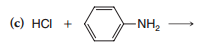# Problem: First, complete and balance each of the equations below. Then, choosing among ethanol, hexane, and liquid ammonia, state which (there may be more than one) might be suitable solvents for each of these reactions. Disregard the practical limitations that come from consideration of “like dissolves like” and base your answers only on relative acidities.

94% (40 ratings)
###### Problem Details

First, complete and balance each of the equations below. Then, choosing among ethanol, hexane, and liquid ammonia, state which (there may be more than one) might be suitable solvents for each of these reactions. Disregard the practical limitations that come from consideration of “like dissolves like” and base your answers only on relative acidities.Frequently Asked Questions

What scientific concept do you need to know in order to solve this problem?

Our tutors have indicated that to solve this problem you will need to apply the Acid Base Equilibrium concept. You can view video lessons to learn Acid Base Equilibrium. Or if you need more Acid Base Equilibrium practice, you can also practice Acid Base Equilibrium practice problems.

What is the difficulty of this problem?

Our tutors rated the difficulty ofFirst, complete and balance each of the equations below. The...as high difficulty.

How long does this problem take to solve?

Our expert Organic tutor, Jonathan took 2 minutes and 22 seconds to solve this problem. You can follow their steps in the video explanation above.

What textbook is this problem found in?

Our data indicates that this problem or a close variation was asked in Organic Chemistry - Solomons 11th Edition. You can also practice Organic Chemistry - Solomons 11th Edition practice problems.